### Home > A2C > Chapter 10 > Lesson 10.1.1 > Problem10-26

10-26.
1. A conveyor belt carries grain to the top of a barn, where it drops the grain so that it forms a pile on the ground. The pile is in the shape of a cone. Recall that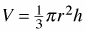is the formula for the volume of a cone. Homework Help ✎

1. Find the volume of the grain when the height of the pile is ten feet and the radius of the base is four feet.

2. The cone-shaped piles during each stage of grain dropping are all similar. If the radius of the base is four feet when the height is ten feet, what is the radius of the base when the height is 15 feet?

3. Consider the angle the side of the cone-shaped pile makes with the ground. Is this angle changing? Justify your answer. If not, what is the measure of the angle?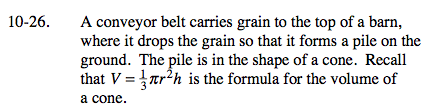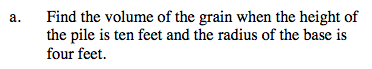$\frac{160\pi}{3}\approx 167.6\text{ cubic feet}$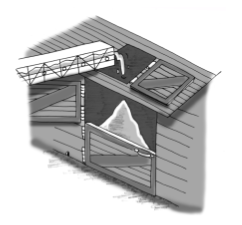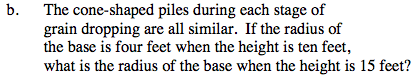6 feet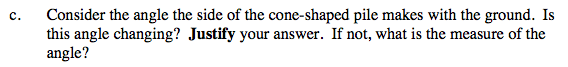Remember that sine, cosine and tangent are all ratios of side lengths. What is true about the angle measures of similar triangles?